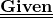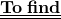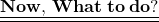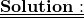## A mobile starts with a speed of 250m / s and begins to decelerate at a rate of 3m / s². How fast is it after 45s?

Question

A mobile starts with a speed of 250m / s and begins to decelerate at a rate of 3m / s². How fast is it after 45s?

in progress 0
4 months 2021-08-09T12:08:41+00:00 1 Answers 4 views 0

1.• Speed of the mobile = 250 m/s
• It starts decelerating at a rate of 3 m/s²
• Time travelled = 45s• Velocity of mobile after 45 secondsWe can solve the above question using the three equations of motion which are:-

• v = u + at
• s = ut + 1/2 at²
• v² = u² + 2as

So, Here a is acceleration of the body, u is the initial velocity, v is the final velocity, t is the time taken and s is the displacement of the body.We are provided with,

• u = 250 m/s
• a = -3 m/s²
• t = 45 s

By using 1st equation of motion,

⇛ v = u + at

⇛ v = 250 + (-3)45

⇛ v = 250 – 135 m/s

⇛ v = 115 m/s

Final velocity of mobile = 115 m/s

━━━━━━━━━━━━━━━━━━━━